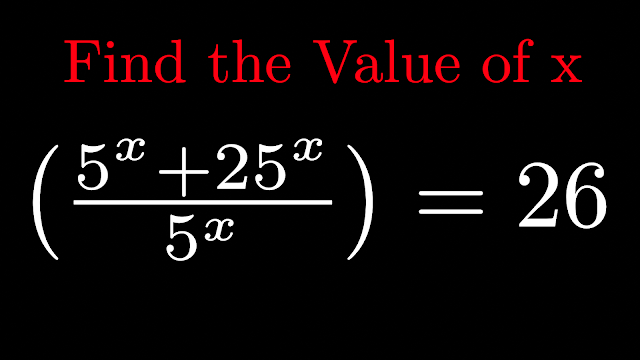# Interesting Math Question from Olympiad-1In this blog we are going to solve this Question

\left( \frac{5^{x}+25^{x}}{5^{x}} \right) = 26

I can Write 25^{x} as 5^{x}\times5^{x} , we get

 \left( \frac{5^{x}+(5^{x}\times 5^{x}) }{5^{x}} \right) = 26

Take 5^{x} common from numerator , we get
 \left( \frac{5^{x}+(1+ 5^{x}) }{5^{x}} \right) = 26

cancelling 5^{x} in numerator and denominator we will get

(1+5^{x}) = 26
5^{x} = 26-1
5^{x}=25
5^{x}=5^{2}
Equating raise to the power we will get
x= 2 that is our answer